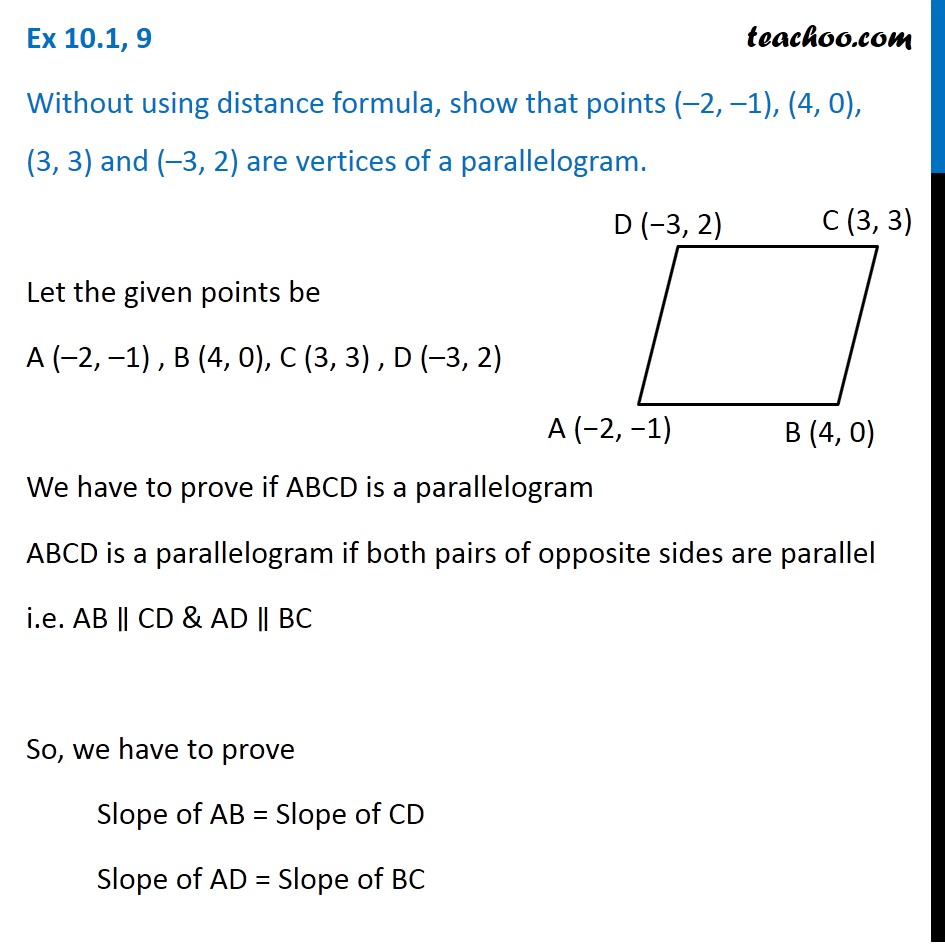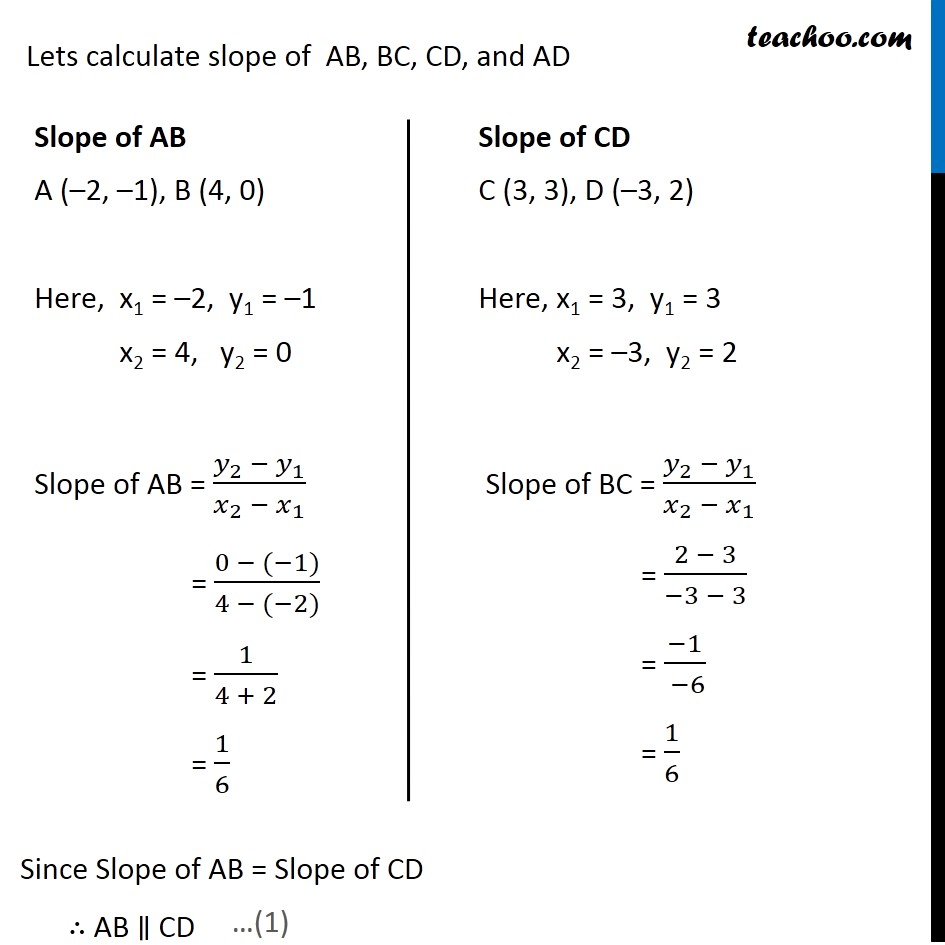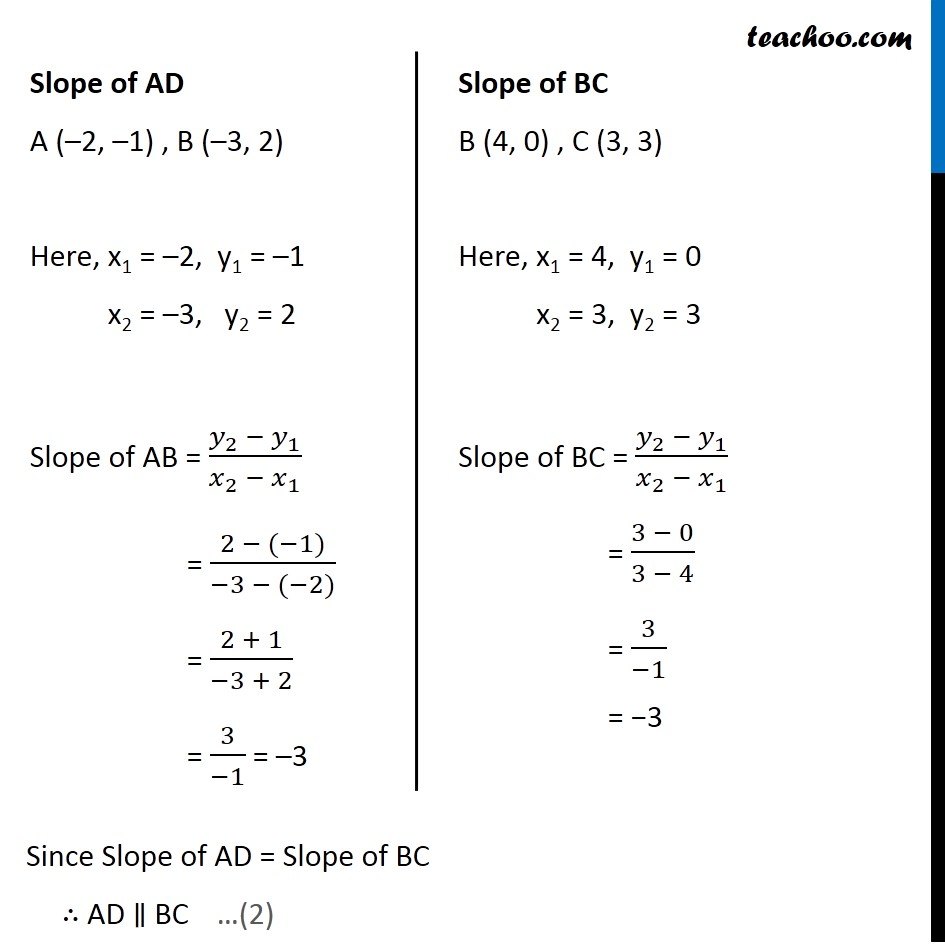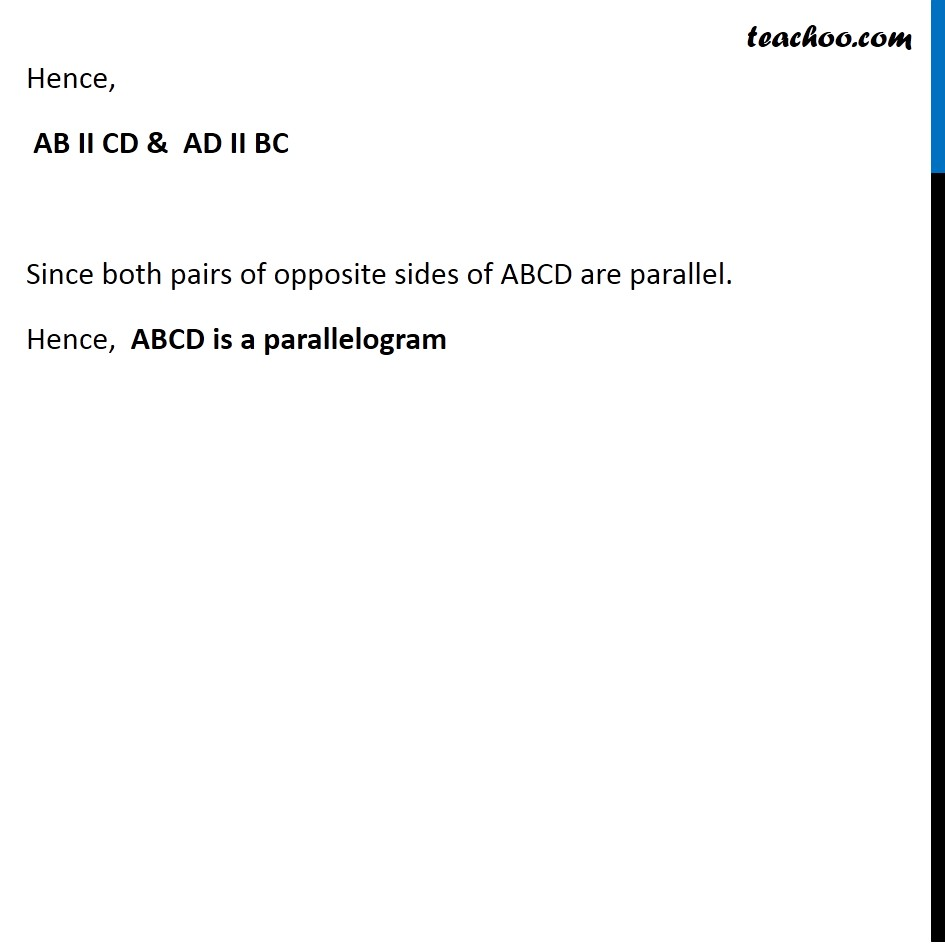Ex 10.1

Chapter 10 Class 11 Straight Lines (Term 1)
Serial order wise### Transcript

Ex 10.1, 9 Without using distance formula, show that points (–2, –1), (4, 0), (3, 3) and (–3, 2) are vertices of a parallelogram. Let the given points be A (–2, –1) , B (4, 0), C (3, 3) , D (–3, 2) We have to prove if ABCD is a parallelogram ABCD is a parallelogram if both pairs of opposite sides are parallel i.e. AB ∥ CD & AD ∥ BC So, we have to prove Slope of AB = Slope of CD Slope of AD = Slope of BC Lets calculate slope of AB, BC, CD, and AD Slope of AB A (–2, –1), B (4, 0) Here, x1 = –2, y1 = –1 x2 = 4, y2 = 0 Slope of AB = (𝑦_2 − 𝑦_1)/(𝑥_2 − 𝑥_1 ) = (0 − (−1))/(4 − (−2)) = 1/(4 + 2) = 1/6 Slope of CD C (3, 3), D (–3, 2) Here, x1 = 3, y1 = 3 x2 = –3, y2 = 2 Slope of BC = (𝑦_2 − 𝑦_1)/(𝑥_2 − 𝑥_1 ) = (2 − 3)/(−3 − 3) = (−1)/( −6) = 1/6 Since Slope of AB = Slope of CD ∴ AB ∥ CD Slope of AD A (–2, –1) , B (–3, 2) Here, x1 = –2, y1 = –1 x2 = –3, y2 = 2 Slope of AB = (𝑦_2 − 𝑦_1)/(𝑥_2 − 𝑥_1 ) = (2 − (−1))/(−3 − (−2)) = (2 + 1)/(−3 + 2) = 3/(−1) = –3 Slope of BC B (4, 0) , C (3, 3) Here, x1 = 4, y1 = 0 x2 = 3, y2 = 3 Slope of BC = (𝑦_2 − 𝑦_1)/(𝑥_2 − 𝑥_1 ) = (3 − 0)/(3 − 4) = 3/(−1) = −3 Since Slope of AD = Slope of BC ∴ AD ∥ BC Hence, AB II CD & AD II BC Since both pairs of opposite sides of ABCD are parallel. Hence, ABCD is a parallelogram